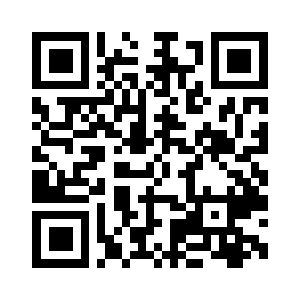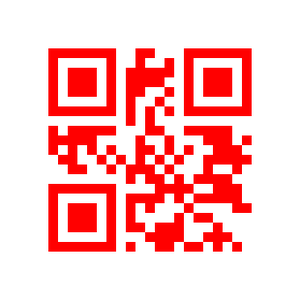Open in App
Not now

# Generate QR Code using qrcode in Python

• Difficulty Level : Basic
• Last Updated : 04 Apr, 2022

A Quick Response Code or a QR Code is a two-dimensional bar code used for its fast readability and comparatively large storage capacity. It consists of black squares arranged in a square grid on a white background.

Python has a library “qrcode” for generating QR code images. It can be installed using pip.

`pip install qrcode`

Approach:

• Import module
• Create Qrcode with qrcode.make() and it returns a PilImage object.
• Save into image

Syntax:

`qrcode.make('Data to be encoded')`

Example 1:

## Python3

 `# Importing library``import` `qrcode` `# Data to be encoded``data ``=` `'QR Code using make() function'` `# Encoding data using make() function``img ``=` `qrcode.make(data)` `# Saving as an image file``img.save(``'MyQRCode1.png'``)`

Output:Example 2:

We can also use QRCode class to create a QR Code and change its details. It takes the following parameters:

• Version: This parameter is an integer from 1 to 40 that controls the size of the QR Code (the smallest, version 1, is a 21×21 matrix).
• error_correction:  This parameter controls the error correction used for the QR Code. There are following four constants available for this :
• qrcode.constants.ERROR_CORRECT_L : About 7% or fewer errors can be corrected.
• qrcode.constants.ERROR_CORRECT_M (default) : About 15% or fewer errors can be corrected.
• qrcode.constants.ERROR_CORRECT_Q: About 25% or fewer errors can be corrected.
• qrcode.constants.ERROR_CORRECT_H: About 30% or fewer errors can be corrected.
• box_size: This parameter controls how many pixels each “box” of the QR code is.
• border: The border parameter controls how many boxes thick the border should be (the default is 4, which is the minimum in the specification).
• add_data(): This method is used to add data to the QRCode object. It takes the data to be encoded as a parameter.
• make(): This method with (fit=True) ensures that the entire dimension of the QR Code is utilized, even if our input data could fit into less number of boxes.
• make_image(): This method is used to convert the QRCode object into an image file. It takes the fill_color and back_color optional parameters to set the foreground and background color.

Below is the implementation:

## Python3

 `# Importing library``import` `qrcode` `# Data to encode``data ``=` `"GeeksforGeeks"` `# Creating an instance of QRCode class``qr ``=` `qrcode.QRCode(version ``=` `1``,``                   ``box_size ``=` `10``,``                   ``border ``=` `5``)` `# Adding data to the instance 'qr'``qr.add_data(data)` `qr.make(fit ``=` `True``)``img ``=` `qr.make_image(fill_color ``=` `'red'``,``                    ``back_color ``=` `'white'``)` `img.save(``'MyQRCode2.png'``)`

Output :My Personal Notes arrow_drop_up